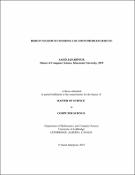## Robust maximum covering location problem (RMCLP)2023
##### Authors
Jafaripour, Saeid
University of Lethbridge. Faculty of Arts and Science
##### Publisher
Lethbridge, Alta. : University of Lethbridge, Dept. of Mathematics and Computer Science
##### Abstract
The Maximum Covering Location Problem (MCLP) is a widely recognized optimization problem used in facility location planning. The objective of this problem is to minimize costs while maximizing accessibility to customers. In this thesis, the MCLP is being solved as an optimization problem under uncertain customer benefits in a network, to minimize regret, which is the difference between the cost of the optimal solution under the worst- case scenario and the cost of the current solution under the worst-case scenario. Three algorithms were implemented to solve the problem and find the optimal solution, including an exact algorithm and two approximate algorithms. The algorithms were evalu- ated using various instances, including the OR-Library and randomly generated instances. The results indicate that the exact algorithm is better at minimizing regret, but it is unable to solve large instances within the allotted time limit. Also, one of the approximate algo- rithms based on a Mean-Scenario, which is a 2-Approximation general algorithm, indicates very competitive results to obtain the Min-Max Regret. The observations of this thesis confirm the results of related research for both the exact algorithm and the Mean-Scenario algorithm, which rely on standard and general methodologies. Also, the solutions obtained by the other approximate algorithm based on randomized rounding are within only nine percent of the Mean-Scenrio algorithm results, which proves the value of this approach.
##### Keywords
Maximum covering location problem , MCLP , Min-max regret , Approximate algorithms , Exact algorithms